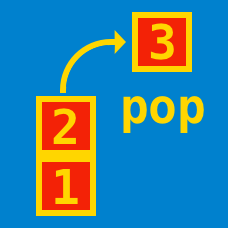Computer Science

# Queues - Basic

The java code below is a simple implementation of an array based data structure that is either a stack or a queue. What type of structure is this, and how should the three methods one(), two(), and three() be renamed so that they describe their functions?

  1 2 3 4 5 6 7 8 9 10 11 12 13 14 15 16 17 18 19 20 21 22 23 24 public class data_structure { private int limit = 1000000; private int[] arr = new int[limit]; private int size = 0; private int rear = limit - 1; public void one(int element){ arr[ rear ] = element; size ++; rear --; } public int two(){ rear ++; int temp = arr[rear]; arr[rear] = 0; size -= 1; return temp; } public int three(){ return arr[rear + 1]; } } 

Suppose the following operations are made on a circular queue structure.

• Insert entry $$A$$.
• Insert entry $$B$$.
• Insert entry $$C$$.
• Remove an entry.
• Remove an entry.
• Insert entry $$D$$
• Insert entry $$E$$.
• Remove an entry.
• Insert entry $$F$$.
• Remove an entry.

After completing these operations, what are the remaining items in the queue?

The code below makes use of a deque object. A deque object is similar to a queue except that it allows the insertion/deletion of an element to both the back and front of the queue. What does the pseudocode below output?

  1 2 3 4 5 6 7 8 9 10 11 12 13 14 15 16 17 18 19 20 21 22 23 24 25 26 27 28 29 30 31 32 function isTrue(string) //Defines a new function 'isTrue' that returns boolean deq = new Deque(); //Creates a new deque object for char in string{ deq.addRear(char) } boolean equal = true //Creates a boolean variable called true while (deq.size() > 1 and equal){ first = deq.removeFront() last = deq.removeRear() if (first != last){ equal = false } return equal boolean value_a = isTrue("radar") boolean value_b = isTrue("plus") boolean value_c = isTrue("madam") if (value_a and value_b and !value_c) print 1 else if (value_a and !value_b and value_c) print 2 else if (!value_a and value_b and value_c) print 3 else if (!value_a and !value_b and value_c) print 4 else if (!value_a and !value_b and !value_c) print 5 else print 6 

The addRear(e) method adds an item to the rear of a deque,the addFront(e) to the front and removeFront() and removeRear() remove the front and rear items of a deque respectively.

Which of the following best represents a queue data structure?

A) A pile of dirty dishes requiring washing

B) Requests on a single shared resource, like a printer

C) A line of people waiting to go to an amusement park ride

D) (B) and (C)

The below is incomplete python code to implement a queue that uses only two int variables(self.size and self.val) to save its contents.

  1 2 3 4 5 6 7 8 9 10 11 12 13 14 15 16 17 from math import log class Integral_queue: def __init__(self): self.val = 0 self.size = 0 def enqueue(self,value): self.val += value * pow(10,self.size) self.size += 1 #def deque(self...) INCOMPLETE def peek(self): return self.val % 10 def is_empty(self): return self.size == 0 

However, this queue only supports single digit integers as elements. Specifically, the only elements that can be pushed to the queue are numbers in the range $$1$$ to $$9$$. The dequeue() method of the queue class is incomplete. Which of the following methods correctly implements the dequeue() method?

A

 1 2 3 4 5 6 def dequeue(self): power = pow(10,self.size-1) ret = self.val / power self.val = self.val % power self.size -= 1 return ret 

B

 1 2 3 4 5 def dequeue(self): ret = self.val % 10 self.val = self.val // 10 self.size -= 1 return ret 

C

 1 2 3 4 5 def dequeue(self): ret = floor(log(val,10)) self.val /= 10 size -= 1 return ret 

D

 1 2 3 4 5 def dequeue(self): ret = int(str(self.val)) self.val = int(str(self.val)[1::]) size -= 1 return ret 

×